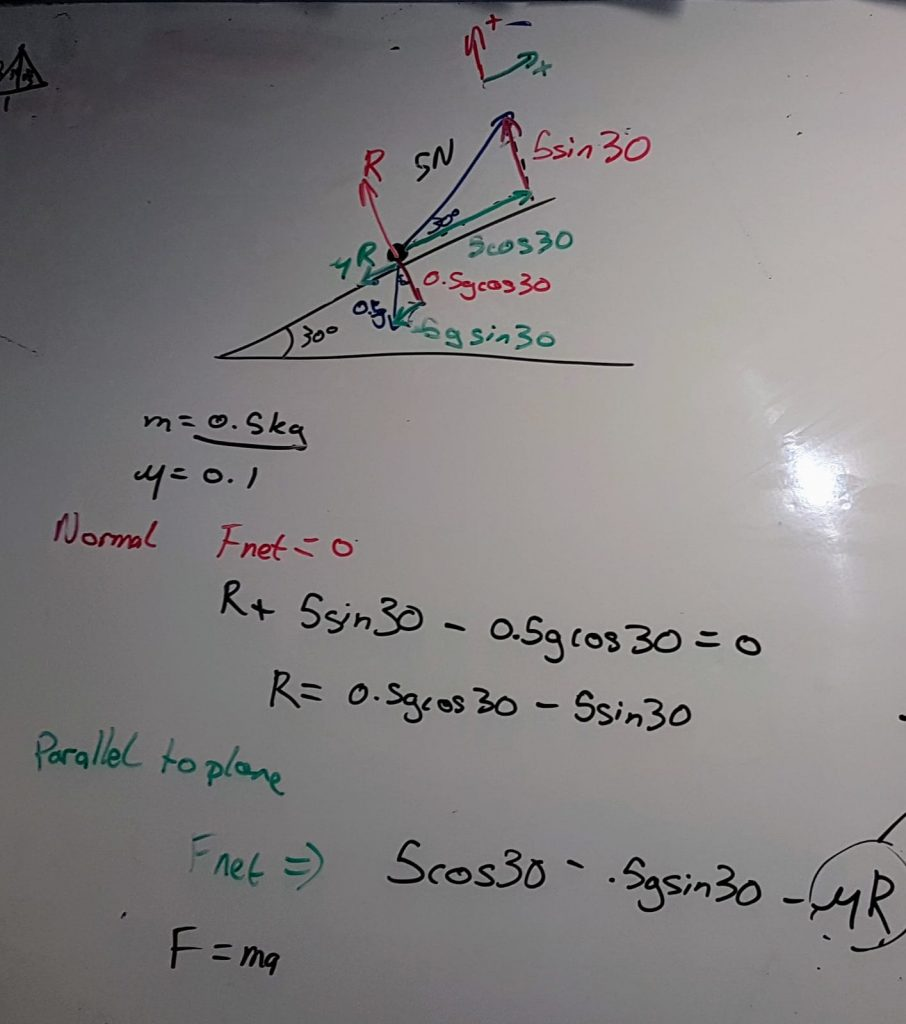# 349. Circumcircle Investigation

The A-level textbook we use has a nice picture of the circumcircle of a triangle and a definition, plus a brief description of how to work through them. For those who are pondering what a circumcircle is, click on the image or link below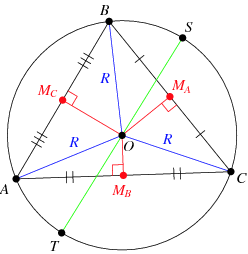Image credit: WolframMathWorld

I’ll just stick to basic vocabulary in this post, rather than the formal circumcentre and circumradius.

Back to the book – not exactly inspiring or memorable stuff!

I looked at the class and off the cuff changed the lesson plan.

Equipment

• Plain paper
• Pencil
• Ruler
• Compasses
• Calculator

Step 1

Draw a decent size triangle on the paper. Label the corners A,B,C.

Step 2

Using geometrical constructions, find the centre of the circle that your triangle fits in. Check by actually drawing the circle

Step 3

Discuss what techniques gave the best results – hopefully you’ll have perpendicular bisectors. There is a nice comparison between bisecting the angles (which some students will do) and bisecting the sides. The angle bisectors always cross inside the triangle, the side bisectors don’t.

Step 4

Randomly generate co-ordinates for A, B, & C. Get the students to pick them and then they can’t moan if the calculations are awful.

Step 5

Discuss how you are going to find the centre and radius of the circumcircle. We decided on:

• Only use two sides
• Find the midpoints
• Generate the equations of the lines through the midpoint
• Find where they intersect
• Use the point and one corner to find the radius

Step 6

Review their methods, looking for premature rounding in questions. I’m still instilling an appreciation for the accuracy of fractions and surds, over reaching for the calculator.

Step 7

This is how my solution looked – I numbered the picture and the steps so students could follow the logic. I was answering on one page projected on screen.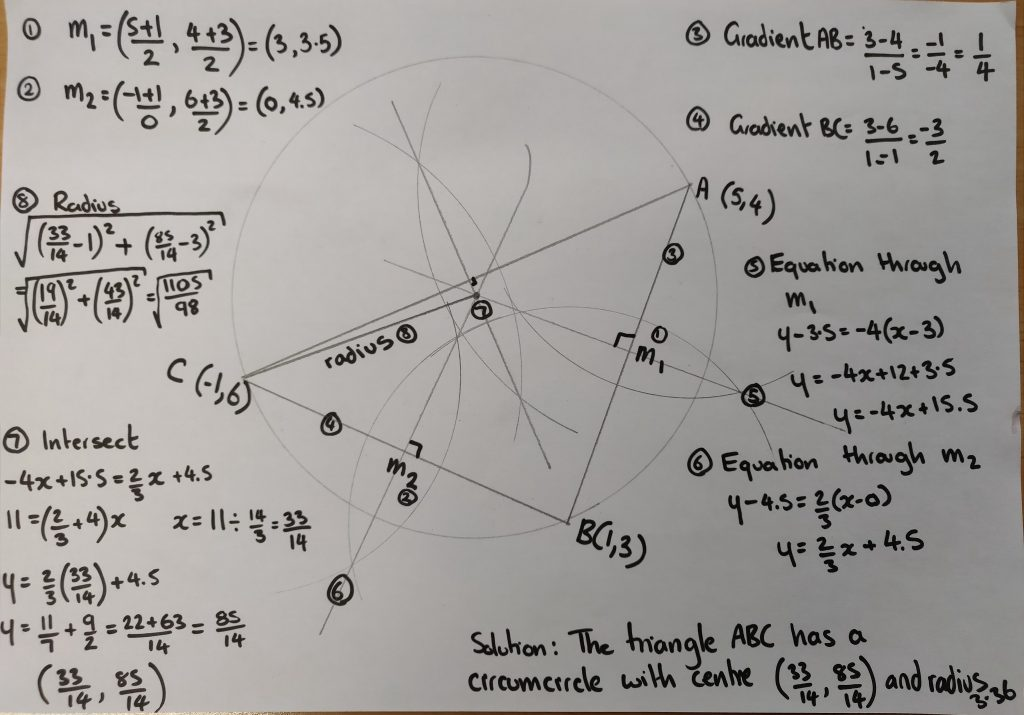# 348. A-Level colouring (Updated)

Those of you who follow this blog will know I have a thing for explaining with colours. This isn’t just a gimmick for younger students, it also works for 16-18 year olds.

In the picture below we were looking at proving a statement involving reciprocal trigonometric functions and fractions. A common source of misconception with this kind of question is that students split the question into working with the numerator and denominator separately, then make mistakes when they put them back together. They can’t see the big picture.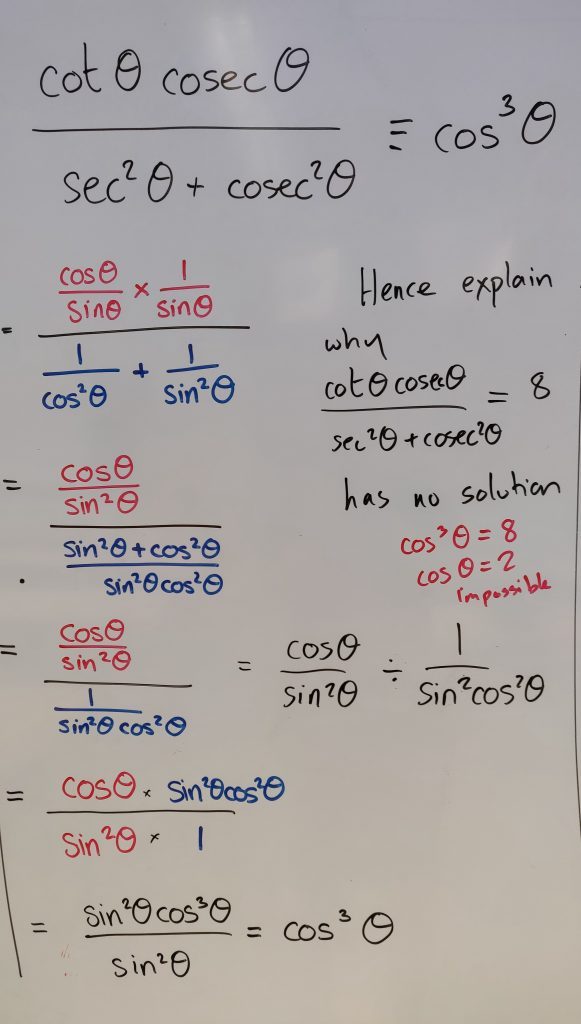Image credit: Mathssandpit

When I discussed this on the board I used separate colours for the expressions in the numerator and denominator. The class could follow the logic so easily. It’s probably my most successful introduction to this topic. I saw that some students used highlighter on their notes after I’d gone through it, so they could track the solution.

The second type of question we looked at was solving a trigonometric equation. The straight forward expansion was all in one colour, but the roots of the quadratic were highlighted in different colours. The reasoning behind this was that students often solve half the quadratic and neglect the other impossible solution. Our exam board likes to see students consider the other solution and formally reject it. It makes the solution complete. By using a colour, the impossible solution stands out and reminds students to provide a whole solution.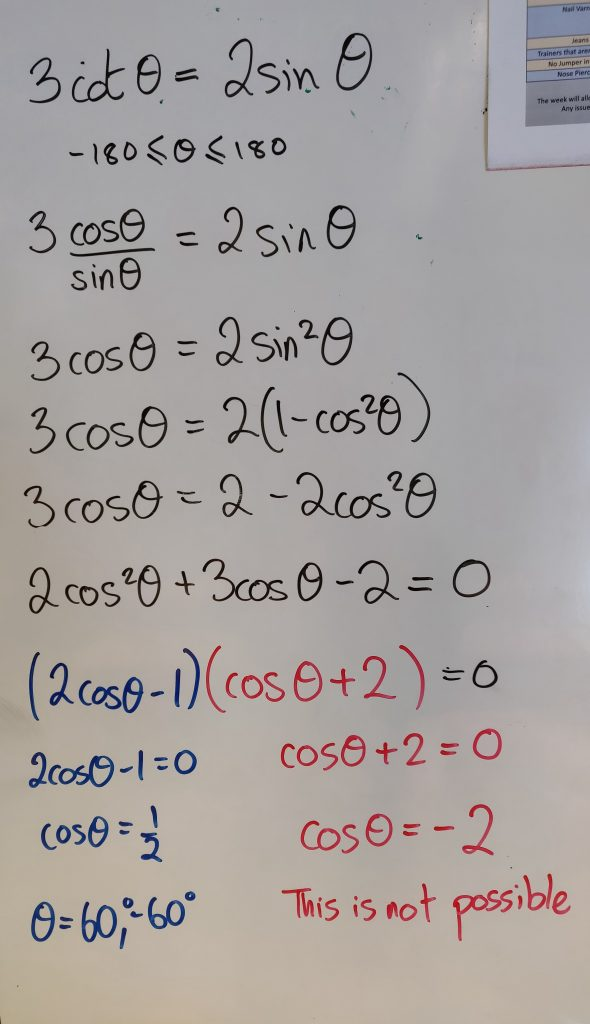Image credit: Mathssandpit

So when you are planning for misconceptions at A-level, remember that coloured pens aren’t just for younger students.

Update: 22nd October

The brilliant Mr B has shared how he uses colour to identify the forces in perpendicular directions in Mechanics.